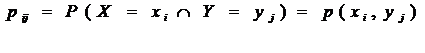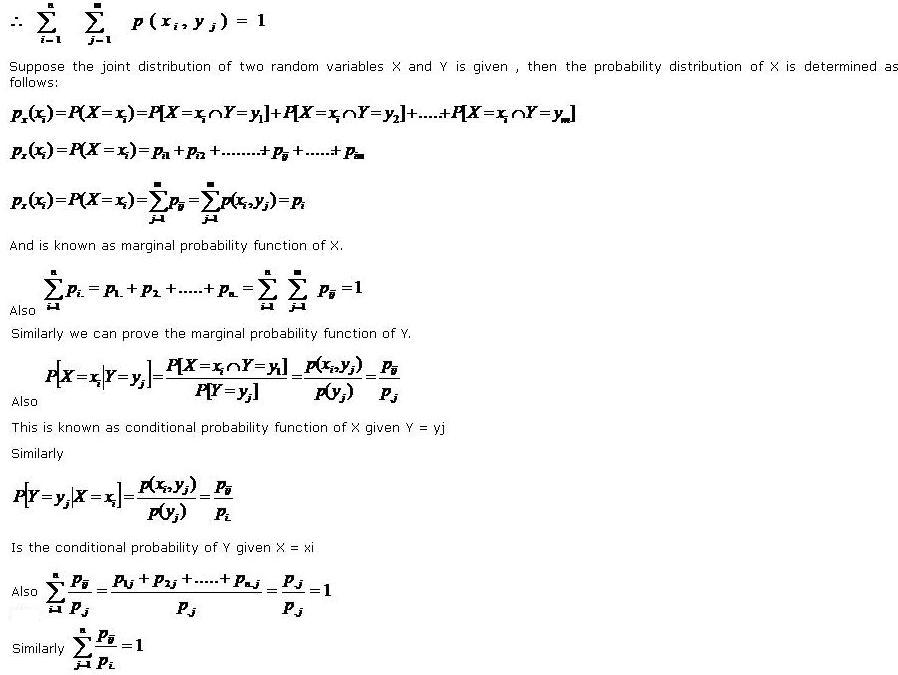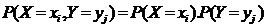# Statistics Assignment Help With Joint Probability Law

## 3.5 Joint Probability Law:

Two random variables X and Y are said to be jointly distributed if they are defined on the same probability space. The sample points consist of 2 tuples. If the joint probability function is denoted by PXY(x,y) then the probability of a certain event E is given by

PXY(x,y) = P[(X,Y) Є E](X,Y) is said to belong to E, if in the 2 dimensional space the 2 tuples lie in the borel set B, representing the event E.

### 3.5.1 Joint probability mass function and marginal and conditional probability function:

Let X and Y be random variables on a sample space S with respective image sets X(S) = { x1, x2,……. xn} and Y(S) ={ y1, y2,……. Ym}. We make the product set

X(S) *Y(S) = { x1, x2,……. xn}*={ y1, y2,……. Ym}

Into a probability space by defining the probability of the ordered pair (xi, yj). the function p on X(S) *Y(S) defined byIs called the joint probability function of X and Y and is usually represented in the form of the following table:

 Y X y1 y2 y3 ...…. yj ……… ym Total x1 p11 p12 p13 …….. p1j ……… p1m p1. x2 p21 p22 p23 …….. p2j …….. p2m p2. x3 p31 p32 p33 …….. p3j …….. p3m p3. . . . . . . . . . . . . . . xi pi1 pi2 pi3 …….. pij …….. pim pj. . . . . . . . . . . . . . . xn pn1 pn2 pn3 …….. pnj …….. pnm pn. Total p.1 p.2 p.3 …….. p.j …….. p.m 1

###Two random variables X and Y are said to be independent ifOtherwise they are said to be independent.